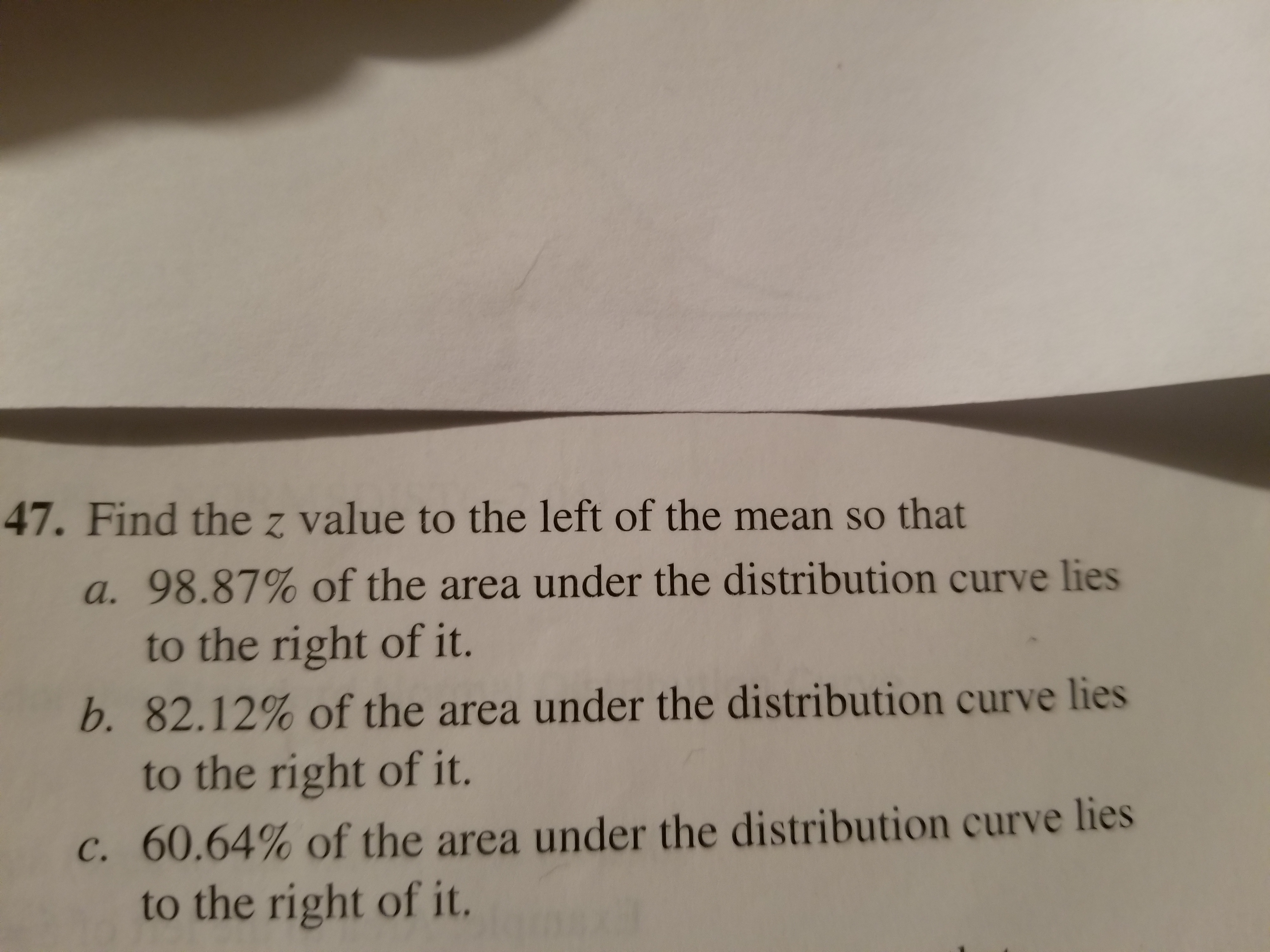# 47. Find the z value to the left of the mean so thata. 98.87% of the area under the distribution curve liesb. 82.12% of the area under the distribution curve liesc, 60.64% of the area under the distribution curve liesto the right of it.to the right of it.to the right of it.

Question
507 viewshelp_outlineImage Transcriptionclose47. Find the z value to the left of the mean so that a. 98.87% of the area under the distribution curve lies b. 82.12% of the area under the distribution curve lies c, 60.64% of the area under the distribution curve lies to the right of it. to the right of it. to the right of it. fullscreen
check_circle

Step 1

The normal distribution:

A continuous random variable X is said to follow normal distribution if the probability density function of X is,

Step 2

The standard normal distribution:

A standard normal variable, the value of which is usually denoted by z, is a normal random variable with mean μ = 0 and standard deviation σ = 1.

Step 3

Calculation for part (a):

The z value to the left of the mean, such that 98.87% or 0.9887 proportion of the ar...

### Want to see the full answer?

See Solution

#### Want to see this answer and more?

Solutions are written by subject experts who are available 24/7. Questions are typically answered within 1 hour.*

See Solution
*Response times may vary by subject and question.
Tagged in

### Statistics